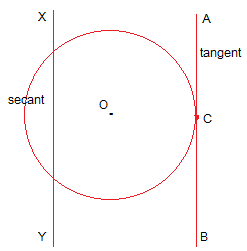## Breaking

CustomWritings.com - get an essay written for you by academic experts. Feel free to get writing assistance online.

## NCERT Solutions for Class 10 Maths Chapter 10 – Circles

Exercise: 10.1

1. How many tangents can a circle have?

A circle can have infinite tangents.

2.  Fill in the blanks :
(i) A tangent to a circle intersects it in ............... point(s).
(ii) A line intersecting a circle in two points is called a .............
(iii) A circle can have ............... parallel tangents at the most.
(iv) The common point of a tangent to a circle and the circle is called ............

(i) one
(ii) secant
(iii) two
(iv) point of contact

3. A tangent PQ at a point P of a circle of radius 5 cm meets a line through the centre O at
a point Q so that OQ = 12 cm. Length PQ is :
(A) 12 cm
(B) 13 cm
(C) 8.5 cm
(D) √119 cmThe line drawn from the centre of the circle to the tangent is perpendicular to the tangent.
∴ OP ⊥ PQ

By Pythagoras theorem in ΔOPQ,
OQ2 = OP2 + PQ2
⇒ (12)= 52 + PQ2
⇒PQ2 = 144 - 25
⇒PQ2 = 119
⇒PQ = √119 cm
(D) is the correct option.

4. Draw a circle and two lines parallel to a given line such that one is a tangent and the
other, a secant to the circle.AB and XY are two parallel lines where AB is the tangent to the circle at point C while XY is the secant to the circle.Courtesy : CBSE